# Examples Of Logic Circuits

By | September 8, 2022

When it comes to understanding how logic circuits work and what kinds of examples are available, it can be confusing for the layperson. Fortunately, there are plenty of resources that can help make sense of it all. Logic circuits are one of the most fundamental components of any digital device, from computers to smartphones, and their applications are broad. In this article, we'll explain what a logic circuit is and then provide some examples of how they are used in everyday technology.

At its core, a logic circuit is a set of interconnected electronic components that performs an operation based on a set of input signals. The output of the logic circuit is either a high or a low voltage or current, depending on the input. These outputs are then used to control other circuit functions, such as turning a light on or off. The logic circuits we’re discussing in this article are digital logic circuits, which use transistors and other components to generate a binary output from an input.

Logic circuits are used in a variety of applications, including in computer systems, robotics, and automated machines. For example, a logic circuit can detect whether a switch is on or off and then trigger an action, such as turning a motor on or off. Similarly, logic circuits are used in most electronics to perform functions like error detection and corrections.

One of the most common types of logic circuits is a logic gate. A logic gate is a basic logic circuit that takes two or more inputs and produces an output according to a set of predetermined conditions. For example, a NAND (not AND) gate will produce a high output if either one or both of the inputs is low. A NOR (not OR) gate will produce a low output if either one or both of the inputs is high. Other types of logic gates include AND, OR, XOR, and Flip-Flops, each of which has its own distinct set of characteristics.

Logic circuits are also used to create complex systems. For instance, a microprocessor is made up of many logic circuits that allow it to interpret and execute instructions. Similarly, a Network Interface Card (NIC) can also be thought of as a logic circuit that connects computers to a network.

Logic circuits are also used in special-purpose applications, such as in medical devices, military equipment, and aerospace technology. For example, logic circuits are used to control the movements of robots, which can perform delicate operations in hazardous environments. Moreover, logic circuits can be used to control the motors and other components of automobiles and aircraft.

In conclusion, logic circuits are a vital component of any digital device and are used in a wide range of applications. They are used to interpret instructions, control motors, and even provide a means of communication between computers and networks. As technology continues to advance, logic circuits will continue to be an integral part of our lives.Circuit Simplification Examples Boolean Algebra Electronics Textbook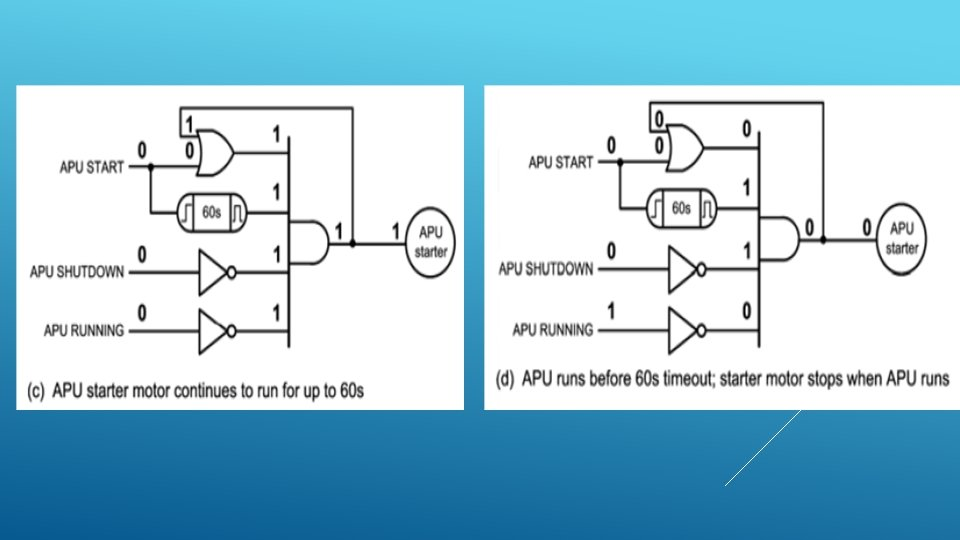Logic Circuits Introduction Aircraft Systems Follow TheI101 Introduction To Informatics Lab 7 Logic CircuitsThe Combinational Logic Circuit Evolved With Proposed System For Scientific Diagram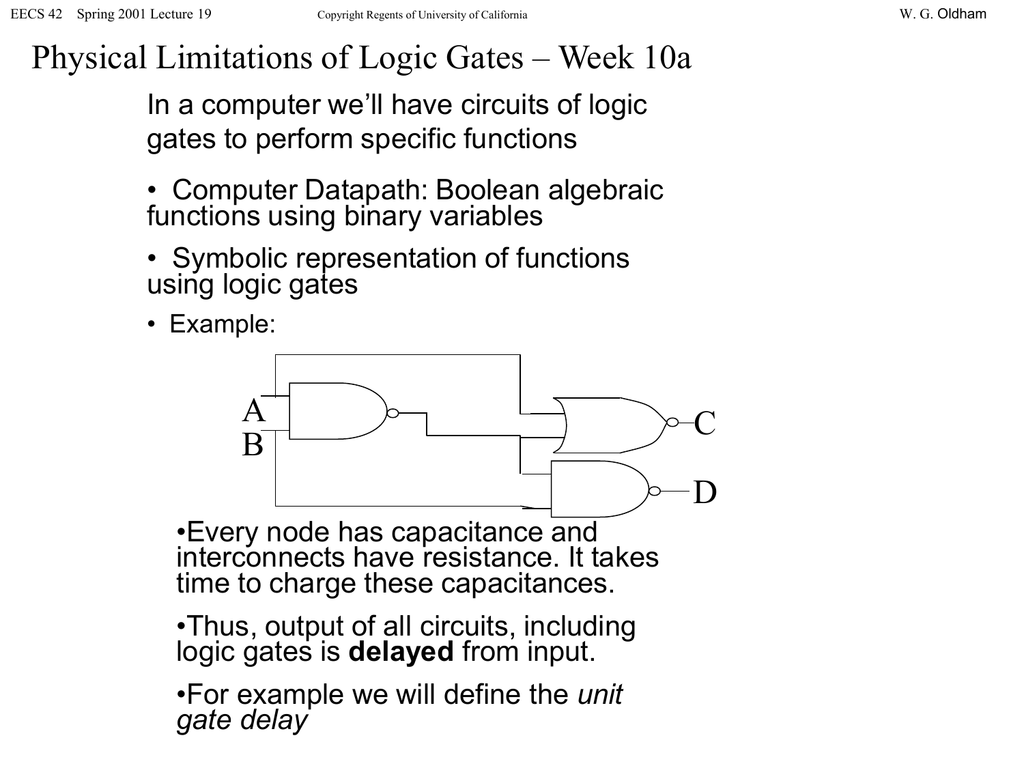Physical Limitations Of Logic Gates Week 10aTypes Of Logic Gates And Their ExamplesLogic Gate Examples Created By The Creately TeamBoolean Algebra Examples Electronics Lab ComSmall Logic Gates The Building Blocks Of Versatile Digital Circuits Part 1 Nuts Volts MagazineExample Logic Circuit 1Logic ExpressionsCombinational Logic CircuitsI101 Introduction To Informatics Lab 7 Logic Circuits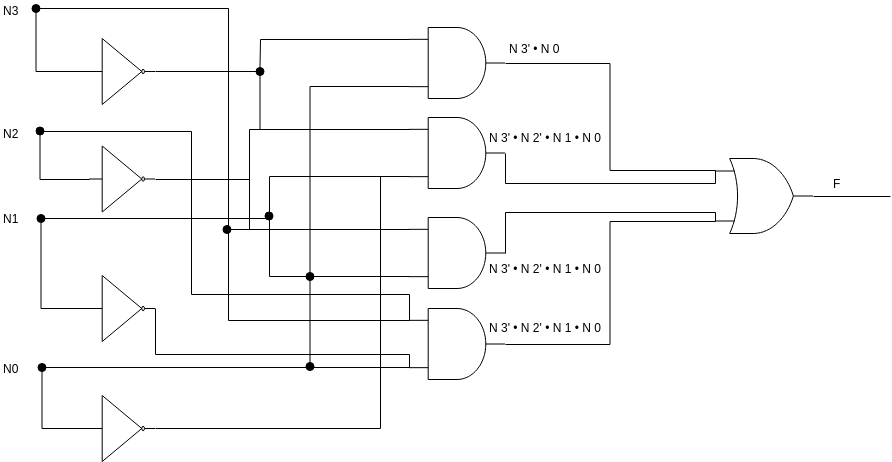Logikdiagramm Software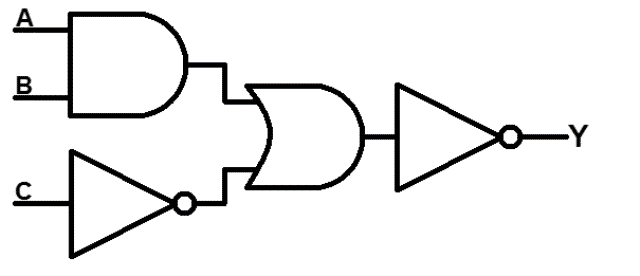Logic Gates Types Working Principle Application Advantage Studymaterialz InDraw A Logic Circuit In Circuitikz Tikzblog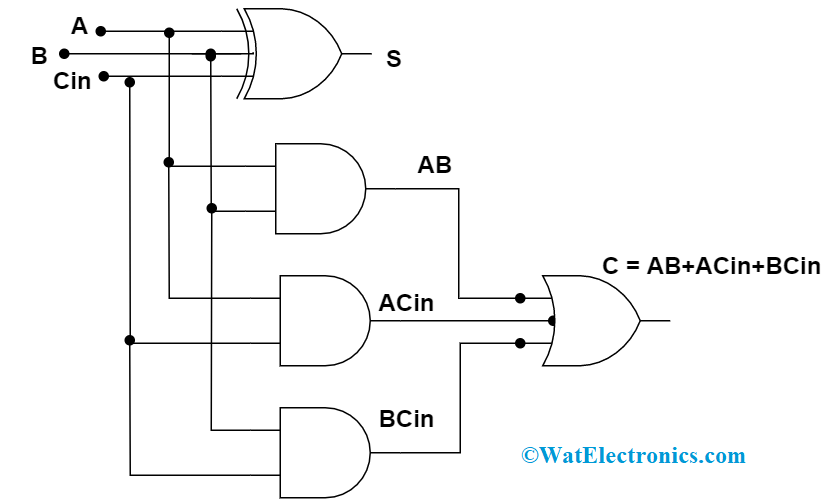Combinational Logic Circuits Definition Examples And ApplicationsExam Questions Logic Gates Bits Of Bytes Co